Jet Set Go! All about Aeroplanes Jet Set Go! All about Aeroplanes

# Tetrahedral Molecular Geometry Questions

Molecular geometry is the study of three-dimensional shapes formed by molecules and how these shapes relate to chemical reactivity and physical properties. The theory of valence shell electron pair repulsion (VSEPR) attempts to explain these electron arrangements’ natural repelling forces.

 Definition: Tetra- means four, and -hedral means the face of a solid; “tetrahedral” literally means “having four faces.” This shape is formed when there are four bonds all on one central atom and no lone electron pairs. According to the VSEPR theory, the bond angles between the electron bonds are 109.5°.

## Tetrahedral Molecular Geometry Chemistry Questions with Solutions

Q-1: Which of the following molecules has tetrahedral geometry?

a) SiH2Br2

b) KrCl2F2

c) PCl5

d) SF4

Explanation: In order to determine the molecular geometry, first calculate the steric number of Si in SiH2Br2.

Steric Number = Number of lone pairs on Si + Number of sigma bond pairs

We know that silicon has 4 valence electrons, and it has made four covalent bonds.

Therefore, number of lone pairs = 0

Number of sigma bond pairs = 4

Steric Number = 4

According to VSEPR theory, a steric number equal to 4 corresponds to tetrahedral molecular geometry.

Q-2: Which carbon in the molecule shown below will have a tetrahedral molecular geometry?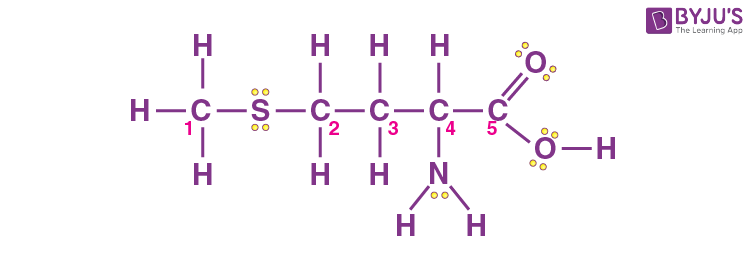a) 1, 2, 3

b) 3, 4, 5

c) 1, 2, 3, 4

d) All of them

Answer: c) 1, 2, 3, 4

Explanation: The carbons 1, 2, 3, and 4 form four sigma bonds and have zero lone pair, giving them a steric number of four. According to VSEPR theory, a steric number of 4 corresponds to tetrahedral molecular geometry.

Q-3: Determine the molecular geometry of the nitrogen atom in the molecule.a) Trigonal planar

b) Tetrahedral

c) Bent

d) Trigonal pyramidal

Explanation: The nitrogen atom in the molecule has three sigma bonds (two with H-atoms and one with C-atoms) and one lone pair. This gives it a steric number of four. According to VSEPR, it will have tetrahedral geometry.

Note: Lone pairs have no effect on the molecule’s molecular geometry. They have an impact on the molecule’s shape.

Q-4: Determine the molecular geometry around each atom in the molecule given below:Atoms

Number of Lone Pairs

Number of Sigma Bond Pairs

Steric Number

Geometry

C-1

0

3

3

Trigonal Planar

N

1

2

3

Trigonal Planar

C-3

0

4

4

Tetrahedral

O

2

2

4

Tetrahedral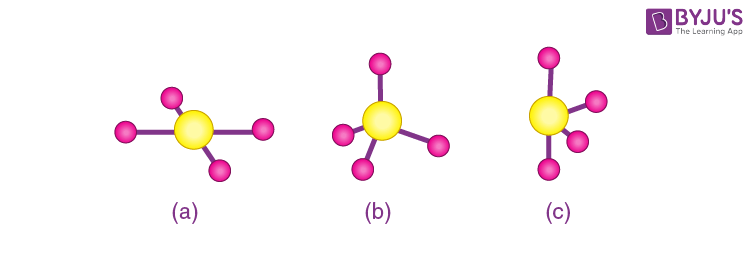Q-5: Identify the shape for each.

a) Square planar

b) Tetrahedral

c) Seesaw

Q-6: Determine the electron domain geometry on which the molecular geometry is based.

a) Octahedral

b) Tetrahedral

c) Trigonal bipyramidal

Q-7: How many lone pairs are on each central atom?

a) Two

b) Zero

c) One

Q-8: Which of the following elements has the potential to be the central atom in shape c)?

Be, C, S, Si

Answer: Because shape (c) has four bonds and only one lone pair, the central atom’s valence shell must have six electrons, which is only possible in the case of S (sulphur).

Q-9: In the following compound, what is the geometry of the nitrogen atom?Answer: Nitrogen forms two sigma bonds and has one lone pair in the given compound. As a result, it will show the hybridisation of sp2 corresponding to trigonal planar geometry.

Q-10: Identify the molecular geometry around the atoms highlighted in red in the given organic molecule.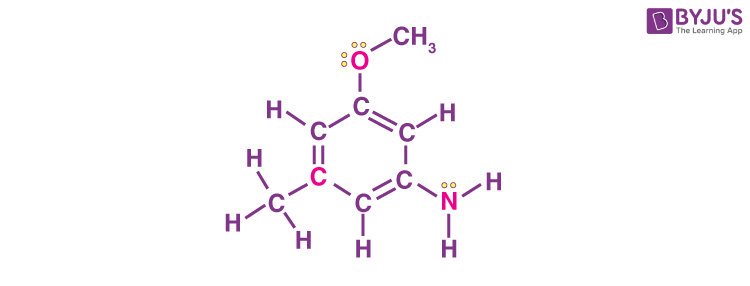Molecular geometry of Carbon: Trigonal Planar

Molecular geometry of Nitrogen: Tetrahedral

Molecular geometry of Oxygen: Tetrahedral

Q-11: What is the electron geometry of the electron groups on the oxygen atom shown below in methanol?Answer: The oxygen atom in the methanol molecule shown has tetrahedral geometry because it has two lone pairs and two bond pairs, corresponding to a steric number of four.

Q-12: Why is it that a tetrahedral complex of the type [MA2B2 ] does not exhibit geometrical isomerism?

Answer: Because all positions of tetrahedral are the same in orientation, a tetrahedral complex of the type [MA2B2 ] does not exhibit geometrical isomerism.

Q-13: [NiCl4]2- is paramagnetic, whereas [Ni(CO)4] is diamagnetic, despite the fact that both are tetrahedral. Why?

Answer: In [NiCl4]2-, Ni has a 3d8 configuration, and Cl is unable to pair up electrons, resulting in a paramagnetic complex. While Ni has a 3d8 4s2 configuration in [Ni(CO)4], CO pairs up electrons. Because all of the electrons in [Ni(CO)4] are paired up, it is diamagnetic.

Q-14: What is the molecular geometry and hybridisation around the carbon atom based on the Lewis dot structure of formic acid shown below?a) Trigonal bipyramidal, sp3d

b) Tetrahedral, sp3

c) Octahedral, sp3d2

d) Trigonal Planar, sp2

Q-15: The shape of [NiF4]2- and [Ni(CN)4]2- respectively are:

a) Square Planar, Square Planar

b) Tetrahedral, Square Planar

c) Tetrahedral, Tetrahedral

d) Square Planar, Tetrahedral

Explanation: Ni is in a +2 oxidation state with a d8 configuration in both coordination complexes. The only difference is that F is a weak field ligand, and CN is a strong field ligand. In the presence of weak field ligands, no pairing occurs, resulting in sp3 hybridisation of Ni in [NiF4]2-, which corresponds to tetrahedral geometry. In the presence of strong field ligands, however, pairing occurs, resulting in dsp2 hybridisation and square planar geometry.

## Practice Questions on Tetrahedral Molecular Geometry

Q-1: Which of the following structures has tetrahedral molecular geometry?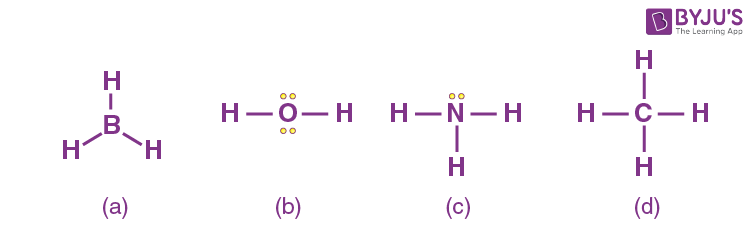Q-2: How many of the atoms in this molecule are tetrahedral?a) 1

b) 2

c) 3

d) 4

Q-3: Consider the following molecule. Determine the molecular geometry and hybridisation of each labelled C-atom.Q-4: An amino acid’s structural formula is shown below. Determine the electron group geometry (EG) and molecular geometry (MG) of the atoms in red.a) C: EG- trigonal planar, MG: trigonal planar; N: Tetrahedral, MG- trigonal pyramidal

b) C: EG- trigonal planar, MG: trigonal pyramidal; N: Tetrahedral, MG- trigonal pyramidal

c) C: EG- trigonal pyramidal, MG: trigonal pyramidal; N: trigonal pyramidal, MG- Tetrahedral

d) C: EG- trigonal pyramidal, MG: trigonal planar; N: Tetrahedral, MG- trigonal pyramidal

Q-5: The chemical structure of fentanyl is shown below. The IUPAC name is N-(1-phenethylpiperidin-4-yl)-N-phenylpropionamide. Give the molecular shape of the numbered atoms.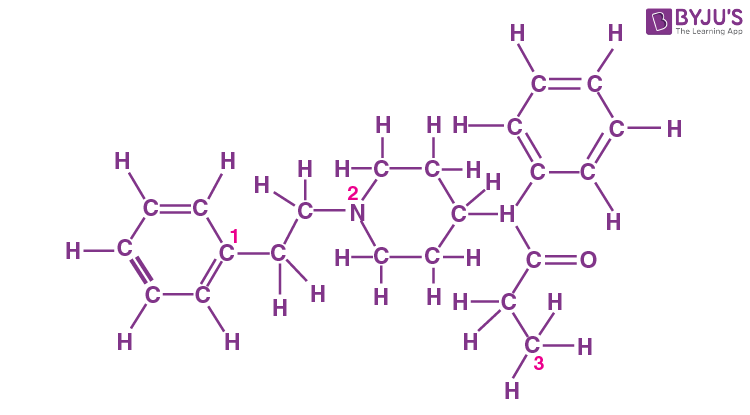Click the PDF to check the answers for Practice Questions.

## Chemical Bonding and Molecular Structure Class 11 Chemistry